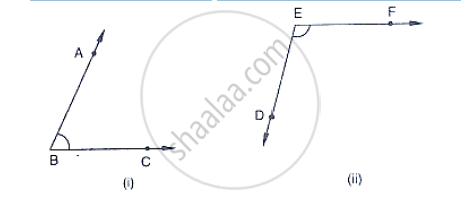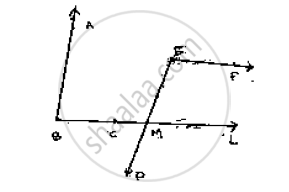# In the Below Fig, Arms Ba and Bc of ∠Abc Are Respectively Parallel to Arms Ed and Ef of ∠Def. Prove that ∠Abc + ∠Def = 180°. - Mathematics

In the below fig, arms BA and BC of ∠ABC are respectively parallel to arms ED and EF of
∠DEF. Prove that ∠ABC + ∠DEF = 180°.#### SolutionGiven AB || DE, BC || EF

To prove: ∠ABC + ∠DEF =180°

Construction: produce BC to intersect DE at M

Proof: Since AB || EM and BL is the transversal

∠ABC = ∠EML          [Corresponding angle]            ……(1)

Also,

EF || ML and EM is the transversal

By the property of co-interior angles are supplementary

∠DEF + ∠EML = 180°

From (i) and (ii) we have

∴∠DEF + ∠ABC = 180°

Concept: Pairs of Angles
Is there an error in this question or solution?

#### APPEARS IN

RD Sharma Mathematics for Class 9
Chapter 10 Lines and Angles
Exercise 10.4 | Q 27 | Page 49